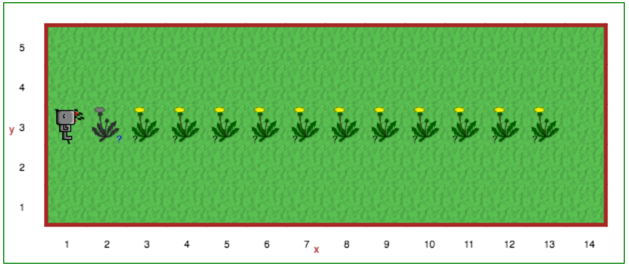# Step 9: Repeating Instructions¶

## Tutorial¶

Often, we will find that we want to repeat a series of instructions a fixed number of times. For example, in Step 8, you had to tell Reeborg to do the same thing over and over again. There is a way in Python to do this … but it has too many new concepts to explain right now. I will just show you the code, and immediately introduce `repeat`, a simpler replacement for it, unique to Reeborg’s World. The standard way is known as a for loop (which we will use later in the course) and is written as follows:

```for i in range(n):
# some
# instructions
# here
```

In Reeborg’s World, we can write a `repeat` loop as follows:

```repeat n:    # where n is a natural number, like 3 or 7
# some
# instructions
# here
```

Note

Using `repeat` will not work in Python programs meant to be run outside of Reeborg’s World.

A loop is a block of instructions that is repeated. Each time the loop is repeated is called an iteration, so the code below would have 4 iterations.

The following code will make Reeborg trace a square:

```repeat 4:
move()
turn_left()
```

By using `repeat`, we can rewrite some function definitions without having to repeat instructions:

```def turn_right():
repeat 3:
turn_left()
```

You might want to adjust the code that you have saved in the library tab to use the repeat block.

For educators

The rationale for having `repeat` as an addition to Python’s standard notation is to avoid having to introduce 4 concepts at the same time (loops, variables as in `_` in `for _ in range(n)`, builtin functions like `range` as well as the concept of function arguments).

By design the `n` in `repeat n` must be an integer literal; it cannot be a variable. When students learn about variables, they should learn the proper Python syntax to do loops and forget about the non-standard `repeat`.Next Section - First Practice Quiz - `repeat`, `if`, `def`